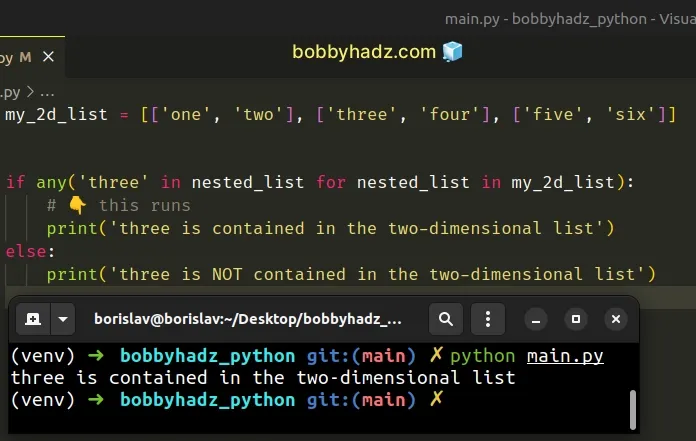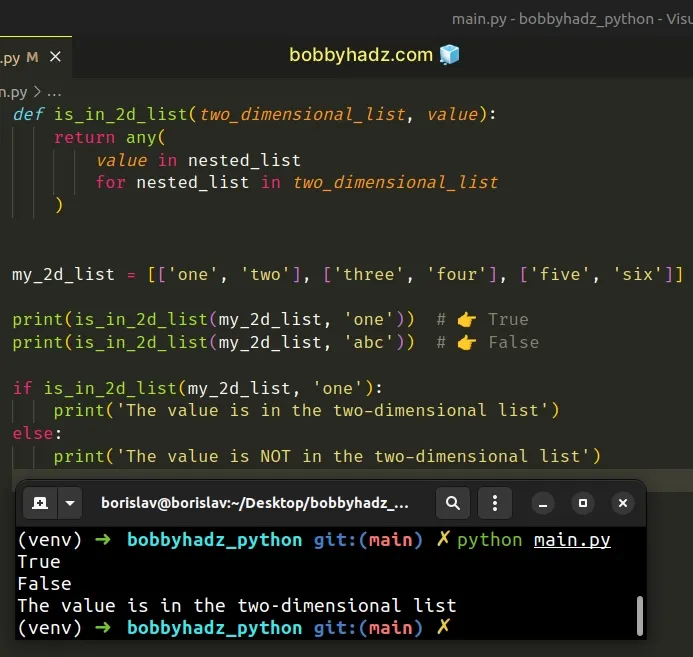# Check if a Value exists in a Two-dimensional List in PythonLast updated: Feb 18, 2023
3 min## #Check if a Value exists in a Two-dimensional List in Python

Use the `any()` function to check if a value exists in a two-dimensional list.

The `any()` function will return `True` if the value exists in the list and `False` otherwise.

main.py
```Copied!```my_2d_list = [['one', 'two'], ['three', 'four'], ['five', 'six']]

if any('three' in nested_list for nested_list in my_2d_list):
# 👇️ this runs
print('three is contained in the two-dimensional list')
else:
print('three is NOT contained in the two-dimensional list')
``````We used a generator expression to iterate over the two-dimensional list.

Generator expressions are used to perform some operation for every element or select a subset of elements that meet a condition.

On each iteration, we check if a specific value is contained in the nested list.

The any function takes an iterable as an argument and returns `True` if any element in the iterable is truthy.

If the specified value is found in any of the nested lists, the `any()` function short-circuits returning `True`.

## #Defining a reusable function

If you have to do this often, define a reusable function.

main.py
```Copied!```def is_in_2d_list(two_dimensional_list, value):
return any(
value in nested_list
for nested_list in two_dimensional_list
)

my_2d_list = [['one', 'two'], ['three', 'four'], ['five', 'six']]

print(is_in_2d_list(my_2d_list, 'one'))  # 👉️ True
print(is_in_2d_list(my_2d_list, 'abc'))  # 👉️ False

if is_in_2d_list(my_2d_list, 'one'):
print('The value is in the two-dimensional list')
else:
print('The value is NOT in the two-dimensional list')
``````The function takes a two-dimensional list and a value as parameters and returns `True` if the value is contained in the two-dimensional list and `false` otherwise.

## #Getting the nested list that contains the value

You can use the assignment expression syntax if you need to get the nested list that contains the specified value.

main.py
```Copied!```my_2d_list = [['one', 'two'], ['three', 'four'], ['five', 'six']]

if any('three' in (match := nested_list) for nested_list in my_2d_list):
# 👇️ this runs
print('three is contained in the two-dimensional list')

print(match)  # 👉️ ['three', 'four']

print(match.index('three'))  # 👉️ 0
else:
print('three is NOT contained in the two-dimensional list')
``````

Assignment expressions allow us to assign to variables within an expression using the `NAME := expression` syntax.

If the iterable is empty or none of the elements in the iterable are truthy, the `any` function returns `False`.

main.py
```Copied!```my_2d_list = [['one', 'two'], ['three', 'four'], ['five', 'six']]

if any('HELLO' in nested_list for nested_list in my_2d_list):
print('HELLO is contained in the two-dimensional list')
else:
# 👇️ this runs
print('HELLO is NOT contained in the two-dimensional list')
``````

None of the nested lists contain an item with the specified value, so the condition is never met and the `any()` function returns `False`.

Alternatively, you can use a for loop.

## #Check if a Value exists in a Two-dimensional List using a `for` loop

This is a three-step process:

1. Use a `for` loop to iterate over the list of lists.
2. Use the `in` operator to check if each sublist contains the value.
3. Break out of the loop once you find a list that contains the value.
main.py
```Copied!```my_2d_list = [['one', 'two'], ['three', 'four'], ['five', 'six']]

exists_in_list = False

for nested_list in my_2d_list:
if 'three' in nested_list:
exists_in_list = True

print(nested_list)

break

if exists_in_list:
print('The value exists in the two-dimensional list')
else:
print('The value does NOT exist in the two-dimensional list')
``````

We used a `for` loop to iterate over the two-dimensional list.

On each iteration, we check if the current sublist contains a given value.

If the value is contained in the sublist, we set the `exists_in_list` variable to `True` and break out of the loop.

The break statement breaks out of the innermost enclosing `for` or `while` loop.

If the value is not contained in the two-dimensional list, the `exists_in_list` variable remains set to `False`.